# Minkowski Scalars

Minkowski scalars are robust and intuitive shape measures. For sufficiently smooth bodies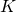, the Minkowski Scalars are just (weighted) integrals over the volume or boundary of the body. There are three linearly independent Minkowski scalars in two dimensions, and four in three dimensions. Minkowski scalars are also known as intrinsic volumes.

The Minkowski scalars can be interpreted as area, perimeter, and the Euler characteristic. The latter is a topological measure; for compact bodies it is given by the number of components minus the number of cavities. All three can be written as integrals, weighted (blue color) by the area, the contour length, and the local curvature of the contour:BodyArea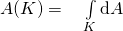Perimeter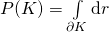Euler characteristic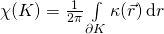where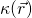is the curvature of the contour at point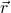. The Euler characteristic is given by the number of components minus the number of cavities:Hadwiger’s characterization theorem  states that any additive, continuous, and motion-invariant functional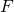of a convex bodycan be expressed as a linear combination of the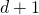Minkowski functionals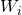of the body:
(1)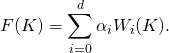Here,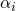are constants independent of. The shape information aboutis completely contained in the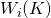, which are,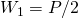, and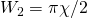. In this sense, the Minkowski scalars are the relevant shape indices for physical functionals with the additivity property.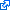H. Hadwiger, Vorlesungen über Inhalt, Oberfläche und Isoperimetrie, Springer-verlag, 1957, vol. 93.